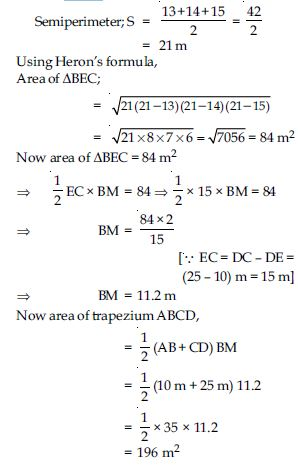# NCERT Solutions for Class 9 Math Chapter 12 - Herons Formula

##### Question 1:

A traffic signal board, indicating ‘SCHOOL AHEAD’, is an equilateral triangle with side ‘a’. Find the area of the signal board, using Heron’s formula. If its perimeter is 180 cm, what will be the area of the signal board?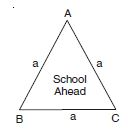(i) Traffic signal board is in the shape of equilateral triangle. Let name it $∆$ABC.
$\therefore$ Side of equilateral triangle ABC = a units (given)
Perimeter of equilateral triangle i.e., 2s = a + a + a = 3a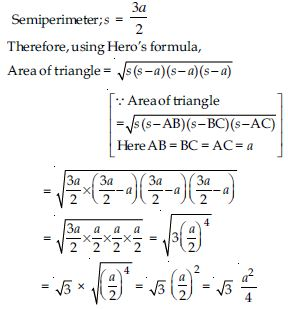(ii) Perimeter of this equilateral triangle = 180 cm
$⇒$ a + a + a= 180 cm
$⇒$ 3a= 180 cm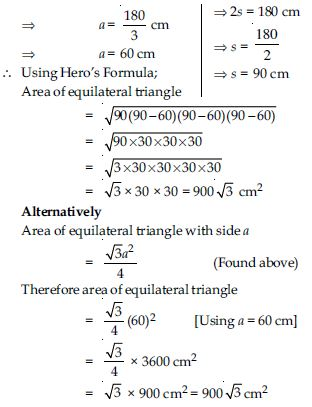##### Question 2:

The triangular side walls of a flyover has been used for advertisements. The sides of the walls are 122 m, 22 m and 120 m (see fig.). The advertisement yield an earning of ` 5000 per m2 per year. A company hired one of its walls for 3 months. How much rent did it pay?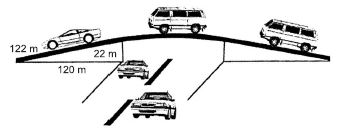Let sides of triangular side walls of fly- over in metres are a, b and c.
$\therefore$ a = 122 m, b = 22 m and c = 120 m
Perimeter of triangle,
2s = (122 + 22 + 120) m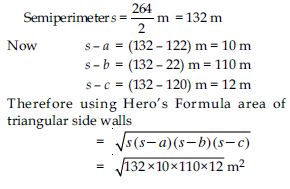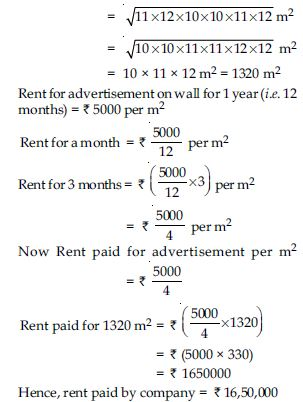##### Question 3:

There is slide in a park. One of its side walls has been painted in some colour with a message ‘‘KEEP THE PARK GREEN AND CLEAN’’, (See Fig.). If the sides of the wall are 15 m, 11 m and 6 m, find the area painted in colour.

Sides of coloured triangular wall are 15 m, 11 m and 6 m.
$\therefore$ Perimeter of this triangular wall;
2s = (15 + 11 + 6)m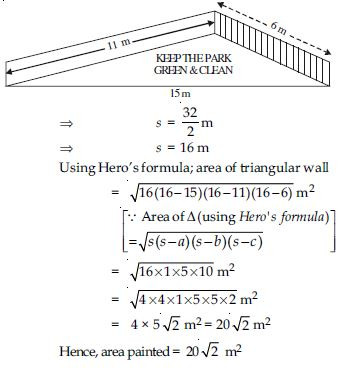##### Question 4:

Find the area of a triangle two sides of which are 18 cm and 10 cm and the perimeter is 42 cm.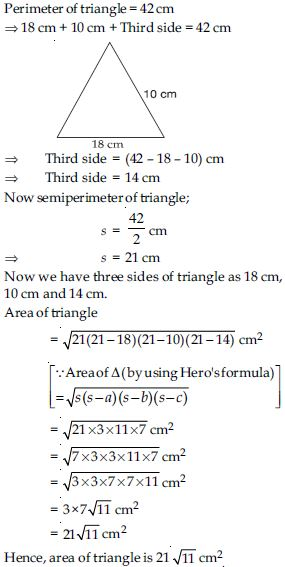##### Question 5:

Sides of a triangle are in the ratio of 12 : 17 : 25 and its perimeter is 540 cm. Find its area.

Given that the sides of triangle are in the ratio of 12 : 17: 25.
$\therefore$ Let sides of triangle in cm are 12x, 17x and 25x.
Perimeter of triangle =540 cm (given)
$⇒$ 12x + 17x + 25x=540 cm
$⇒$ 54x=540 cm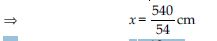$⇒$ x =10 cm
Now sides of triangle are
12x = 12 × 10 = 120 cm
17x = 17 × 10 = 170 cm
and 25x = 25 × 10 = 250 cm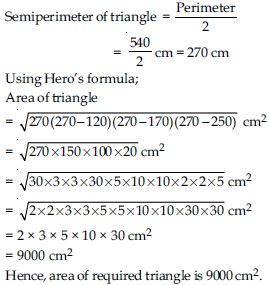##### Question 6:

An isosceles triangle has perimeter 30 cm and each of the equal sides is 12cm. Find the area of the triangle.

Perimeter of an isosceles triangle = 30 cm
$⇒$12 cm + 12 cm + Third side = 30 cm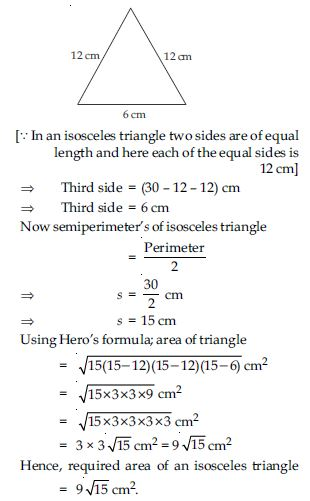##### Question 7:

A park, in the shape of a quadrilateral ABCD, has ∠C = 90°, AB = 9 m, BC = 12 m, CD = 5 m and AD = 8 m. How much area does it occupy?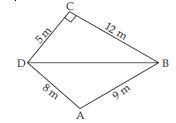In Fig., if we join
BD, we observe that diagonal BD divides quadrilateral ABCD in two triangles (i) Right triangle BCD and (ii) $∆$ABD.
In rt. $∆$BCD, rt. angle is at C.
Therefore Base; CD = 5 m
and Altitude, BC = 12 m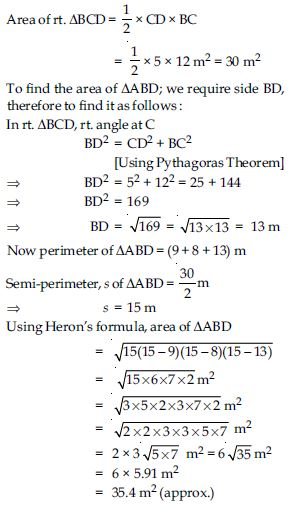= Area ($∆$BCD) + Area ($∆$ABD)
= 30 m2 + 35.4 m2
= (30 + 35.4) m2
= 65.4 m2
Hence, area occupied by the quadrilateral is 65.4 m2.

##### Question 8:

Find the area of a quadrilateral ABCD in which AB = 3 cm, BC = 4 cm, CD = 4 cm, DA = 5 cm and AC = 5 cm.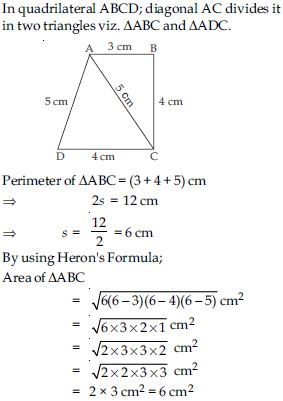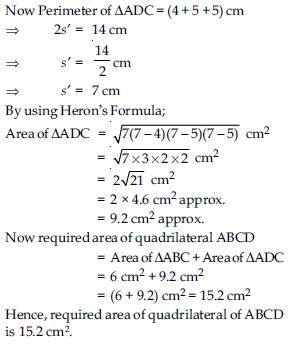##### Question 9:

Radha made a picture of an aeroplane with coloured paper as shown in Fig. Find the total area of the paper used.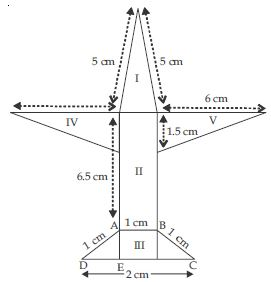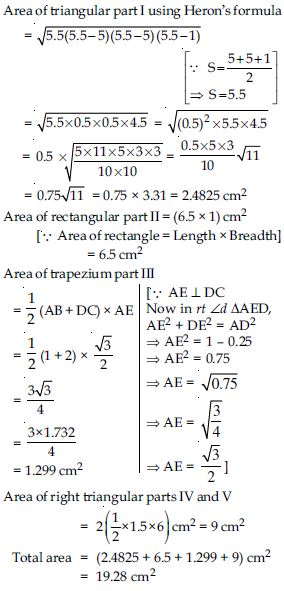##### Question 10:

A triangle and a parallelogram have the same base and the same area. If the sides of the triangle are 26 cm, 28 cm and 30 cm, and the parallelogram stands on the base 28cm, find the height of the parallelogram.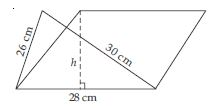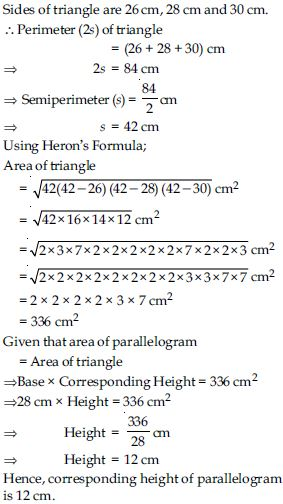##### Question 11:

A rhombus shaped field has green grass for 18 cows to graze. If each side of the rhombus is 30 m and its longer diagonal is 48 m, grass of how much area of grass field will each cow be getting?

Let rhombus shaped field be ABCD.
Therefore its each equal side;
AB = BC = CD = DA
= 30 m
and let its longer diagonal AC = 48 m, Diagonal
AC divides the rhombus in two congruent triangles.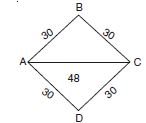(i) $∆$ABC and (ii) $∆$ACD. As we know that area of two congruent triangles are equal.
Therefore area ($∆$ABC) = area ($∆$ACD)
Now it is enough to find the area of $∆$ABC whose sides are AB = BC = 30 m and AC = 48 m Perimeter (2s) of $∆$ABC = (30 + 30 + 48) m
$⇒$ 2s = 108 m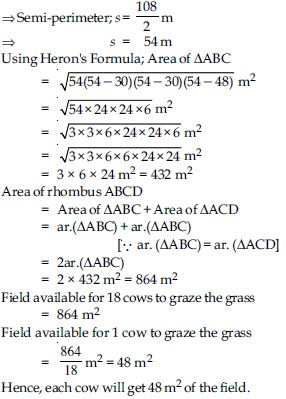##### Question 12:

An umbrella is made by stitching 10 triangular pieces of cloth of two different colours (See Fig.) each piece measuring 20 cm, 50 cm and 50 cm. How much cloth of each colour is required for the umbrella?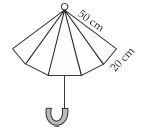Sides of each of 10 triangular pieces of two different colours are 20 cm, 50 cm and 50 cm.##### Question 13:

A kite is in the shape of a square with a diagonal 32 cm and an isosceles triangle of base 8 cm and sides 6 cm each is to be made of three different shades as shown in fig.How much paper of each shade has been used in it ?

Let ABCD be a square whose each sides is a cm
and; diagonal
AC = BD = 32 cm
In rt. $∆$ABC,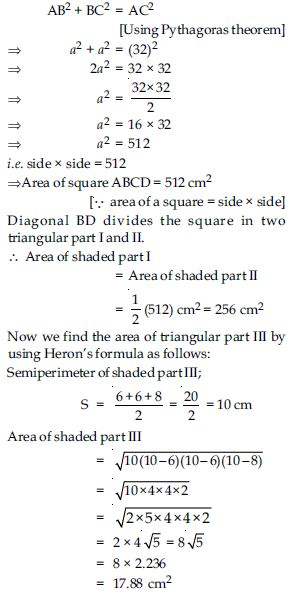##### Question 14:

A floral design on a floor is made up of 16 tiles which are triangular, the sides of the triangle being 9 cm, 28 cm and 35 cm (See Fig.). Find the cost of polishing the tiles at the rate of 50 p per cm2.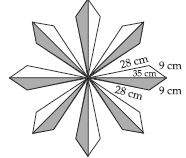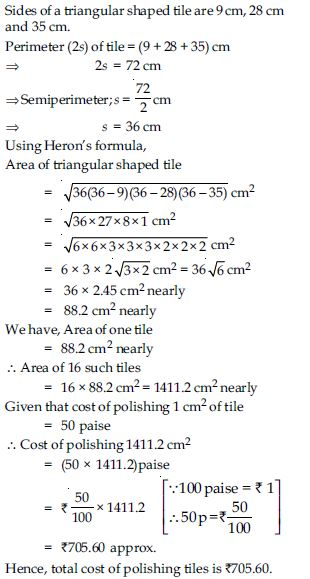##### Question 15:

A field is in the shape of a trapezium whose parallel sides are 25 m and 10 m. The nonparallel sides are 14 m and 13 m. Find the area of the field.

Let ABCD be a field in the shape of trapezium.
Parallel sides AB = 10 m
and CD = 25 m
and Non-parallel sides; AD = 13 m
and BC = 14 m
Draw BM $\perp$ DC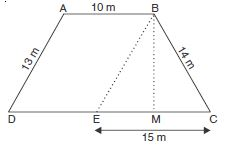also draw BE || AD so that ABED is a
parallelogram
$\therefore$ BE = AD = 13 m
and DE = AB = 10 m
Now in $∆$BEC,